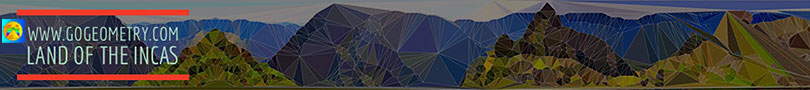Problem 145. Four Triangles, Incircle, Tangent and Parallel to Side, Incenters, Circumcenters.
 In the figure below, given a triangle ABC and the incircle of center I (inscribed circle), DE, FG, and HM are tangent to the incircle I and  parallel to AC, AB, and BC respectively. O, O1, O2, and O3 are the circumcenters of triangles ABC, AHM, BDE, and CFG respectively. I1, I2, and I3 are the incenters of triangles AHM, BDE, and CFG respectively. If d = OI, d1 = O1I1, d2=O2I2, and O3 = O3I3, (1) prove that d, d1, d2, and d3 are parallel, (2) prove that d = d1 + d2 + d3. View or post a solution.Home | Geometry | Search | Problems | 141-150 | Email | By Antonio Gutierrez